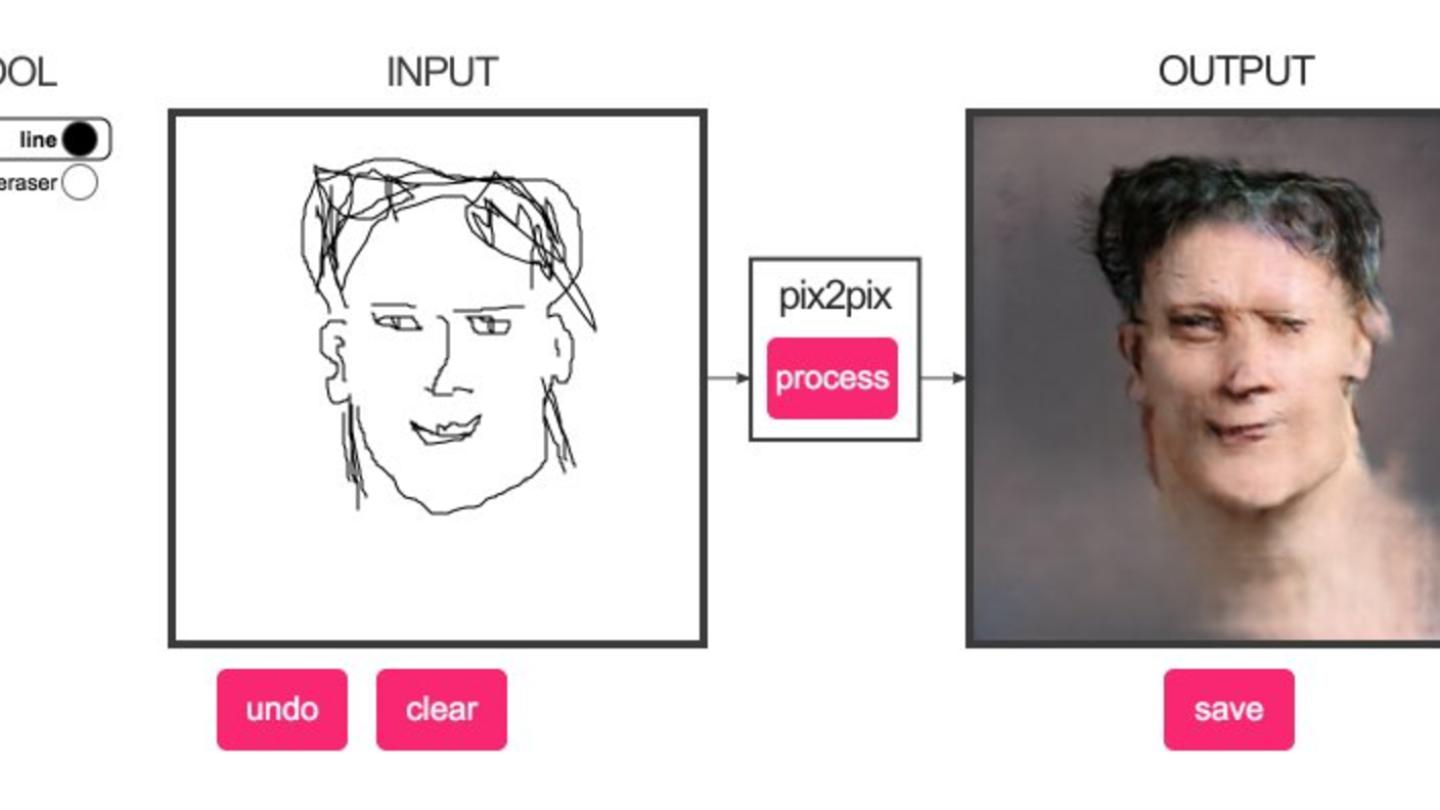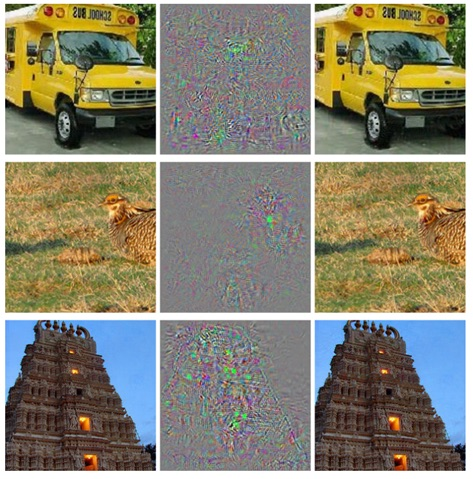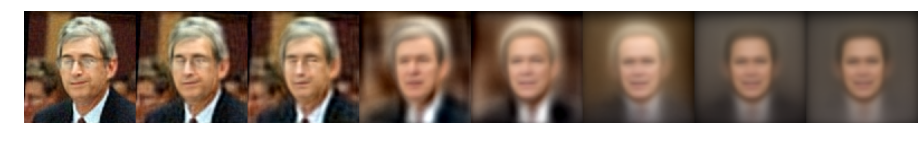# Nightmare Eigenface

Alex Egg,

This post was inspired by the pix2pix project (http://fotogenerator.npocloud.nl) which uses Generative adversarial networks (GANs) to color outlines of faces w/ quite hellish results. A tech blogger dubbed it “Nightmare Hellface Generator is Cutting-Edge Machine Learning” (https://motherboard.vice.com/en_us/article/nightmare-hellface-generator-is-cutting-edge-machine-learning) which I though was hilarious and thus in homage to that I named this.

I wanted to explore an introduction to Generative Models, so here is a famous example called Eigenfaces. This is a classic technique from computer vision and a first step towards understanding generative models.## Introduction to GANs

According to Yann LeCun, these networks could be the next big development. For example, let’s consider a trained CNN that works well on ImageNet data. Let’s take an example image and apply a perturbation, or a slight modification, so that the prediction error is maximized. Thus, the object category of the prediction changes, while the image itself looks the same when compared to the image without the perturbation. From the highest level, adversarial examples are basically the images that fool ConvNets.Figure 1: The images in the left most column are correctly classified examples. The middle column represents the distortion between the left and right images. The images in there right most column are predicted to be of the class ostrich. Even though the difference between the images on the left and right is imperceptible to humans, the Convolutional Network makes classification errors.

Adversarial examples (paper) definitely surprised a lot of researchers and quickly became a topic of interest. Now let’s talk about the generative adversarial networks. Let’s think of two models, a generative model and a discriminative model.

The discriminative model has the task of determining whether a given image looks natural (an image from the dataset) or looks like it has been artificially created. The task of the generator is to create natural looking images that are similar to the original data distribution. This can be thought of as a zero-sum or minimax two player game. The analogy used in the paper is that the generative model is like “a team of counterfeiters, trying to produce and use fake currency” while the discriminative model is like “the police, trying to detect the counterfeit currency”. The generator is trying to fool the discriminator while the discriminator is trying to not get fooled by the generator. As the models train through alternating optimization, both methods are improved until a point where the “counterfeits are indistinguishable from the genuine articles”.

Why It’s Important

According to Yann LeCun, “adversarial training is the coolest thing since sliced bread”. The pioneering work on GANs can be attributed to Ian Goodfellow in 2014.

The basic idea of these networks is that you have 2 models, a generative model and a discriminative model.

Sounds simple enough, but why do we care about these networks? As Yann LeCun stated in his Quora post, the discriminator now is aware of the “internal representation of the data” because it has been trained to understand the differences between real images from the dataset and artificially created ones. Thus, it can be used as a feature extractor that you can use in a CNN. Plus, you can just create really cool artificial images that look pretty natural to me (link).

## Eigenfaces

You’ll need the following python packages and the IFW faces dataset: http://vis-www.cs.umass.edu/lfw/lfw-funneled.tgz

%matplotlib inline

import fnmatch
import os
import matplotlib.pyplot as plt
from PIL import Image
import numpy as np
from tqdm import tqdm
import random
from sklearn.decomposition import PCA

lfw_path = "./data/lfw_funneled/"

images = []
for root, dirnames, filenames in os.walk(lfw_path):
for filename in fnmatch.filter(filenames, '*.jpg'):
images.append(os.path.join(root, filename))

n = len(images)
print("loaded %d face images" % n)

loaded 13233 face images

ids = np.random.randint(n, size=8)

img = [Image.open(images[id]) for id in ids] #choose 8 random images and load into memory
img = np.concatenate([np.array(im.getdata()).reshape((im.size, im.size, 3)) for im in img ], axis=1)
plt.figure(figsize=(16,2))
plt.axis('off')
_=plt.imshow(Image.fromarray(img.astype('uint8')))#resize images
#and put them in 2d matrix (interlace pixels)
w = h = 100

X = np.zeros((n, w*h*3))
for i, img in tqdm(enumerate(images)):
im = Image.open(img)
im = im.resize((w, h))#.convert('L')
pixels = list(im.getdata())
X[i, :] = np.array(pixels).flatten()

13233it [01:45, 125.04it/s]

n_components = 100
pca = PCA(n_components=n_components, svd_solver='randomized', whiten=True)
pca.fit(X)

PCA(copy=True, iterated_power='auto', n_components=100, random_state=None,
svd_solver='randomized', tol=0.0, whiten=True)

#project original data onto PCA components
Xp = pca.transform(X)

#reconstruction
Xt = pca.inverse_transform(Xp)
Xt = np.clip(Xt, 0, 255)

img_pairs = [[X[idx].reshape((w,h,3)), Xt[idx].reshape((w,h,3))] for idx in ids]
img_all = np.concatenate([ np.concatenate([p, p]) for p in img_pairs], axis=1)
plt.figure(figsize=(16,4))
plt.axis('off')
_=plt.imshow(Image.fromarray(img_all.astype('uint8')))What has essentially happened, is that we learned the base representation of the dataset in the matrix $U$ which are the principal component vectors. We have effectively introduced compression by only selecting 100 of the original 256.

We then used the base representation $U$ and the reduced dataset $X’$ to reconstruct X. As you can see there is some loss in quality.

We have created reduced version of our original dataset using the Eigen decomposition of our face dataset. We can now reconstruct the original dataset using the inverse operation:

Where $\hat{X}$ is the lossy reconstruction of the original X using principal components $U$ and the reduced data $X^{‘}$

## Synthetic Faces (Generator)

You’ll remember, from the introduction to GANs above, we described a Generator, well here is an example of a simple generator that uses our principal components + random noise to create creepy synthetic faces!

However, what if we do the reconstruction w/ something else, other than $X^{‘}$? In theory, $U$ holds the principle components that make up a face. Let’s try a reconstruction w/ random numbers and see what happens:

Y = [ np.random.normal(size=n_components) for i in range(8) ]
Yt = [ np.clip(pca.inverse_transform(y), 0, 255) for y in Y ]
Yt = np.concatenate([ yt.reshape((w,h,3)) for yt in Yt ], axis=1)
plt.figure(figsize=(16,2))
plt.axis('off')
plt.imshow(Image.fromarray(Yt.astype('uint8')))These are completely synthetic faces generated from the base model for a face which is described in $U$.

## Base Decomposition

Lets see how PCA generalized the faces of our dataset.

npca = [2000, 1000, 500, 100, 50, 10, 2, 1]
Xt = []
for p in npca:
print("computing PCA for %d components"%p)
pca = PCA(n_components=p, svd_solver='randomized', whiten=True)
pca.fit(X)
Xp = pca.transform(X)
Xt.append(np.clip(pca.inverse_transform(Xp), 0, 255))

computing PCA for 2000 components
computing PCA for 1000 components
computing PCA for 500 components
computing PCA for 100 components
computing PCA for 50 components
computing PCA for 10 components
computing PCA for 2 components
computing PCA for 1 components

idxs = ids
for i,idx in enumerate(idxs):
img_orig = X[idx].reshape((w,h,3))
img_reconstructed = np.concatenate([Xt[p][idx].reshape((w,h,3)) for p in range(len(npca))], axis=1)
plt.figure(figsize=(16,2))
plt.axis('off')
plt.imshow(Image.fromarray(img_reconstructed.astype('uint8')))As you can see all of our images decompose down to the abstract figure of a white male w/ short dart hair wearing a suit. This is a product of imbalance in our original dataset.

As you can see PCA a good method for finding the salient features in high dimensional data. But as we shall see we can do much better for generative models by using nonlinear methods like variational auto-encoders or generative adversarial networks instead.